# Gas Laws – Boyle’s, Charles, Gay Lussac, Avogadro and Ideal Gas Law

## What is a Perfect Gas?

A perfect gas or an ideal gas is a state of a substance, whose evaporation from the liquid state is completed and which obeys all the gas laws strictly under all conditions of pressure and temperature. In reality there is no ideal or perfect gas which obeys all the law of gas under all conditions of pressure and temperature. But the real gases such as oxygen, hydrogen, nitrogen and air which are ordinary difficult to liquefy; within certain range of pressure and temperature may be considered as perfect gas (or ideal gas)

The physical properties of a gas is controlled by three variables and these are

1. Pressure exerted by the gas,
2. Volume occupied by the gas and
3. Temperature of the gas.

The behavior of a perfect gas can be studied with the following gas laws that are proved from experimental results.

1. Boyles Law
2. Charles’s Law
3. Gay-Lussac Law

Let’s discuss these laws of gas in detail

## Boyle’s Law

This law was established by Robert Boyle in 1662.

Statement:

It states that under constant temperature, the absolute pressure of a given mass of a perfect gas varies inversely as its volume.

MathematicallySuffixes 1, 2 and3 … represents different sets of conditions.

Explanation:

For understanding this law lets take an experiment.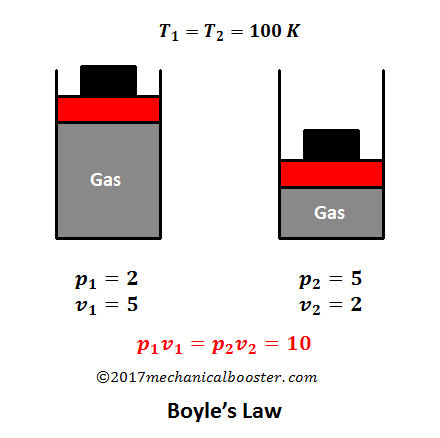Suppose we have a cylinder piston assembly which contains a gas. Keeping this system at room temperature (so the temperature remains constant), If we decrease the volume by applying an external force than the pressure of the gas inside the cylinder increases. And if the external load is removed than the volume of the gas starts increasing and pressure will decreases. This proves that when pressure of a perfect gas increases than its volume will decrease and when volume decreases then pressure increases.

## Charles’s Law

This law was given in 1787, by a French man Jacques A.C. Charles.

#### Statement

This law can be stated in two ways

Statement 1

At constant absolute pressure, the volume of a given mass of a perfect gas varies directly to its temperature.

Explanation: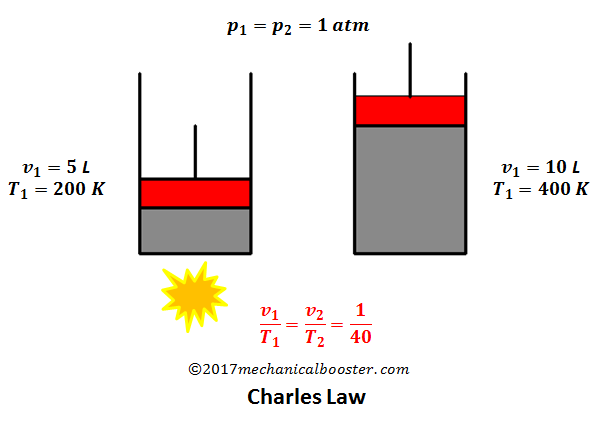Let us again take a piston cylinder assembly which is kept at room temperature. The upper part of the piston is in exposed to the atmospheric pressure (so the system remains at constant absolute pressure). The lower portion of the cylinder is made conductor of heat. Now heat the cylinder form bottom. After some time the piston of the cylinder starts moving upward. Why this has happen? Can you guess? Let me tell you what has actually happened. When the cylinder is heated, temperature of the gas inside the cylinder increases and also increases the pressure which makes the gas expand and exerts a force on the piston and makes it move upward. The piston moves upward to keep the pressure constant (i.e. inside pressure of the gas becomes equal to the atmospheric pressure). The upward movement of the piston increases the volume of the gas

So we can conclude that when a system is at constant absolute pressure, at that time the volume of the gas varies directly to its temperature.

Mathematically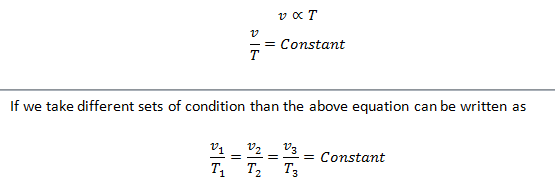Statement 2

At constant pressure, all the prefect gases changes the volume by 1/273th of its original volume at 0 degree Celsius for every 1 degree Celsius change in temperature.

Let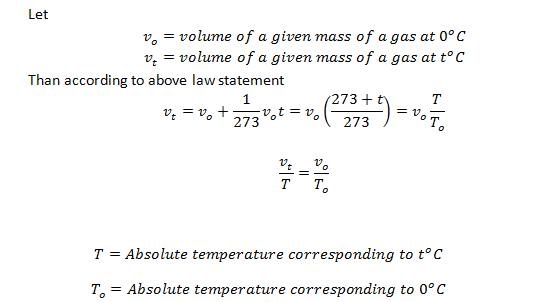#### What is absolute zero temperature?

Since the volume of the gas decreases by 1/273 th of its original volume at 0 degree C by every 1 degree decrease in temperature. So if we take the temperature -273 o C, then the volume of the gas reduces to zero.

The temperature at which the volume of a gas becomes equals to zero is called absolute zero temperature.

## Gay-Lussac’s Law

This law was given by Joseph Louis Gay-Lussac in 1809.

Statement

At constant volume, the absolute pressure of a given mass of a perfect gas varies directly at its absolute temperature.

MathematicallyWhere suffixes 1,2 and 3 … represents different sets of conditions.

Explanation: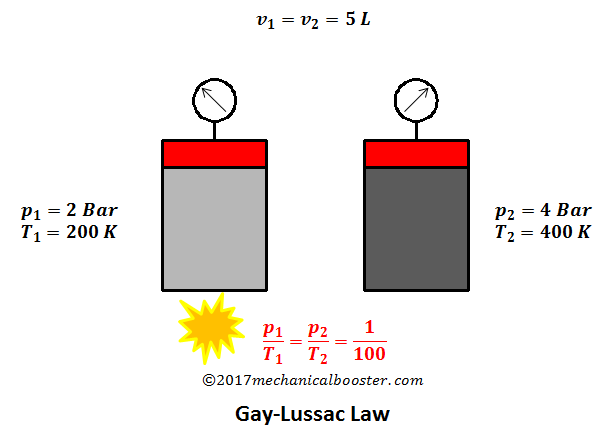let’s take a piston cylinder assembly having an ideal gas in it. And the piston is not movable. Since the piston is fixed, so the volume is constant. Now add some heat to the gas present in the cylinder. The temperature of the gas starts increasing and increases the pressure of the gas. The increase in the pressure can be seen on the pressure gauge attached to the system.

The above experiment proves that at constant volume, the pressure of a perfect gas varies directly to its temperature.

Avogadro law is sometime also called as Avogadro hypothesis or Avogadro’s principle. It is an experimental law which was given in 1811 by Amedeo Avogadro.

Statement:

Under constant temperature and pressure, equal volumes of all the gases contains equal no of molecules.

Or

For a given mass of an ideal gas, the volume of the gas is directly proportional to the amount of substance (moles) of gas, given that the temperature and pressure are constant.

Mathematically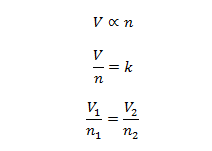Suffixes 1,2 represents different sets of conditions for a gas.

Where

V = volume of the gas.
n = the amount of substance of the gas (in moles).
k = constant which is equals to RT/P, where R is the universal gas constant, T is temperature in Kelvin and P is the pressure. Since the temperature and pressure is constant, so RT/P is also constant and represented as k.

## 5. General Gas Equation or Ideal Gas Law

Ideal gas law or general gas equation is the combined form of boyles law, Charles law and Avogadro law. The ideal gas equation is written asWhere

ρ = Density of the gas = m/V

P = pressure of the gas
V = Volume of the gas
n = Amount of substance of gas (in moles)
R= Ideal, or universal gas constant
T = Absolute temperature of the gas

Summary of all the three Gas Laws (i.e. Boyle’s law, Charles Law and Gay-Lussac law) are given below: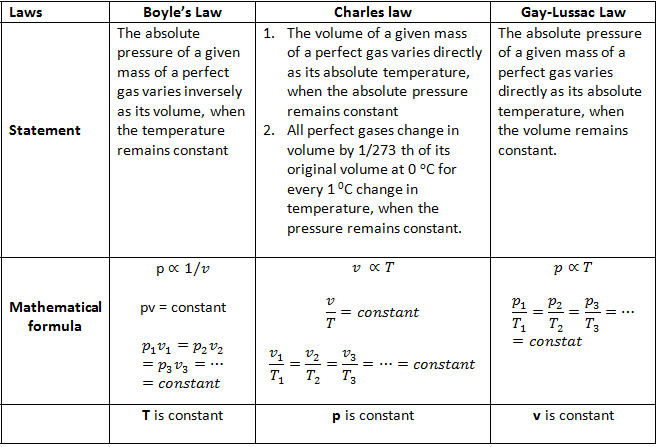If you find any difficult in understanding these gas Laws, you can ask me your problem through comments. If you find this topic informative than share it for others.